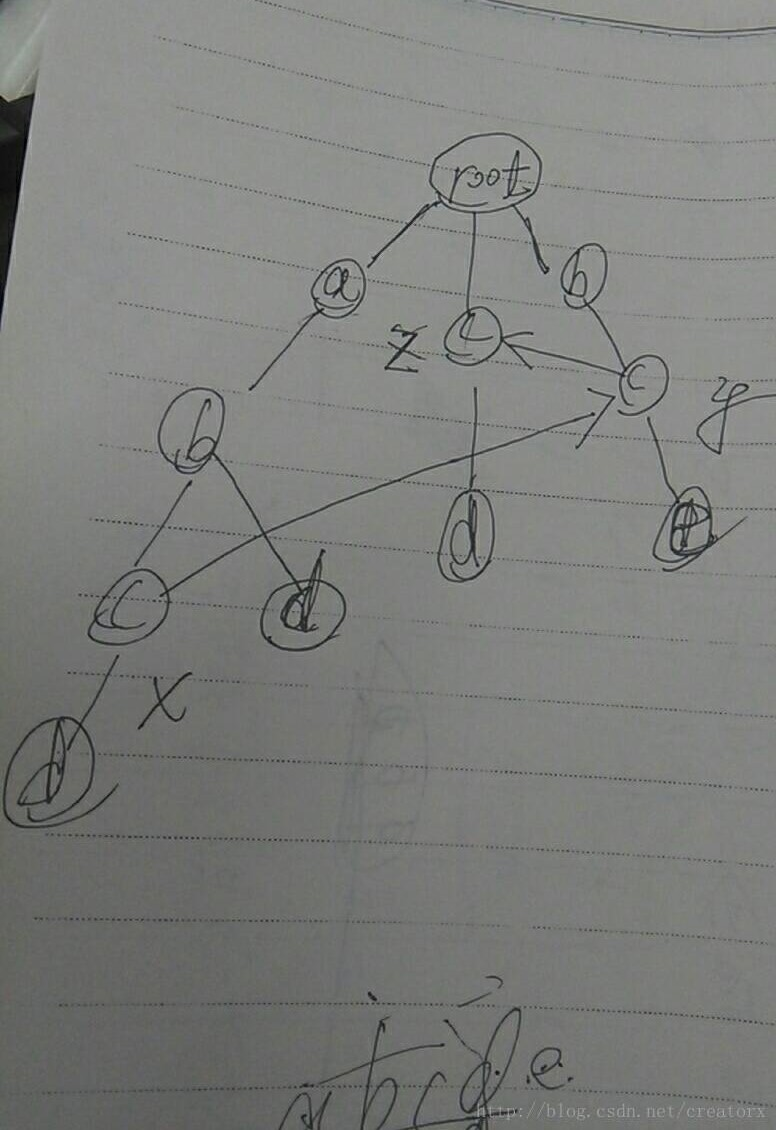# ac自动机最详细的讲解，让你一次学会ac自动机。

1.把所有的单词建立一个字典树。

struct node{
node *next;
node *fail;
int sum;
};


sum是这个节点是不是一个单词的结尾，以及相应的个数。

void Insert(char *s)
{
node *p = root;
for(int i = 0; s[i]; i++)
{
int x = s[i] - 'a';
if(p->next[x] == NULL)
{
newnode=(struct node *)malloc(sizeof(struct node));
for(int j=0;j<26;j++) newnode->next[j] = 0;
newnode->sum = 0;newnode->fail = 0;
p->next[x]=newnode;
}
p = p->next[x];
}
p->sum++;
}void build_fail_pointer()
{
tail = 1;
node *p;
node *temp;
{
for(int i = 0; i <= 25; i++)
{
if(temp->next[i])
{
if(temp == root)
{
temp->next[i]->fail = root;
}
else
{
p = temp->fail;
while(p)
{
if(p->next[i])
{
temp->next[i]->fail = p->next[i];
break;
}
p = p->fail;
}
if(p == NULL) temp->next[i]->fail = root;
}
q[tail++] = temp->next[i];
}
}
}
}


void ac_automation(char *ch)
{
node *p = root;
int len = strlen(ch);
for(int i = 0; i < len; i++)
{
int x = ch[i] - 'a';
while(!p->next[x] && p != root) p = p->fail;
p = p->next[x];
if(!p) p = root;
node *temp = p;
while(temp != root)
{
if(temp->sum >= 0)
{
cnt += temp->sum;
temp->sum = -1;
}
else break;
temp = temp->fail;
}
}
}


#include<bits/stdc++.h>
using namespace std;
const int maxn = 1e7 + 5;
const int MAX = 10000000;
int cnt;
struct node{
node *next;
node *fail;
int sum;
};
node *root;
char key;
node *q[MAX];
node *newnode;
char pattern[maxn];
int N;
void Insert(char *s)
{
node *p = root;
for(int i = 0; s[i]; i++)
{
int x = s[i] - 'a';
if(p->next[x] == NULL)
{
newnode=(struct node *)malloc(sizeof(struct node));
for(int j=0;j<26;j++) newnode->next[j] = 0;
newnode->sum = 0;newnode->fail = 0;
p->next[x]=newnode;
}
p = p->next[x];
}
p->sum++;
}
void build_fail_pointer()
{
tail = 1;
node *p;
node *temp;
{
for(int i = 0; i <= 25; i++)
{
if(temp->next[i])
{
if(temp == root)
{
temp->next[i]->fail = root;
}
else
{
p = temp->fail;
while(p)
{
if(p->next[i])
{
temp->next[i]->fail = p->next[i];
break;
}
p = p->fail;
}
if(p == NULL) temp->next[i]->fail = root;
}
q[tail++] = temp->next[i];
}
}
}
}
void ac_automation(char *ch)
{
node *p = root;
int len = strlen(ch);
for(int i = 0; i < len; i++)
{
int x = ch[i] - 'a';
while(!p->next[x] && p != root) p = p->fail;
p = p->next[x];
if(!p) p = root;
node *temp = p;
while(temp != root)
{
if(temp->sum >= 0)
{
cnt += temp->sum;
temp->sum = -1;
}
else break;
temp = temp->fail;
}
}
}
int main()
{
int T;
scanf("%d",&T);
while(T--)
{
root=(struct node *)malloc(sizeof(struct node));
for(int j=0;j<26;j++) root->next[j] = 0;
root->fail = 0;
root->sum = 0;
scanf("%d",&N);
getchar();
for(int i = 1; i <= N; i++)
{
gets(key);
Insert(key);
}
gets(pattern);
cnt = 0;
build_fail_pointer();
ac_automation(pattern);
printf("%d\n",cnt);
}
return 0;
}



05-16227

#### 强势 图解 AC自动机（保证您一次就能学会！）

10-053万+

#### AC自动机 算法详解(图解)及模板03-302万+

#### AC自动机总结

11-238万+

#### AC自动机算法

07-192万+

#### 最详细的讲解，让你一次学会主席树

03-162857

#### 多模式匹配算法：AC自动机的C++实现

06-142714

#### AC快乐机——最最通俗易懂的AC自动机讲解！

04-1259

#### 【AC自动机（有了这个我就能AC了吗）】

06-111471

#### AC自动机字符串匹配——python代码实现

08-016418

#### AC自动机

04-293155

#### AC自动机的python实现

07-221979

#### AC自动机的优化及经典例题

07-176414

#### AC自动机总结（超详细注释）

08-20169

#### ac自动机

05-02107

#### AC自动机讲解超详细

09-163万+

#### Aho-Corasick算法学习

06-301837

#### 初级玩转Linux+Ubuntu(嵌入式开发基础课程)

06-26

06-103万+

#### 我以为我对Mysql事务很熟，直到我遇到了阿里面试官©️2020 CSDN 皮肤主题: 大白 设计师: CSDN官方博客点击重新获取扫码支付1.余额是钱包充值的虚拟货币，按照1:1的比例进行支付金额的抵扣。
2.余额无法直接购买下载，可以购买VIP、C币套餐、付费专栏及课程。余额充值